• No products in the cart.

# 301.2.6-How Map Reduce Works

### How MapReduce Works

• We will understand MapReduce with the help of an example
• Word count example
• Imagine a huge data set that contains user comments and discussions about a laptop
• We want to count the frequency of words to get an idea on the popular features

#### Map Reduce Word Count Example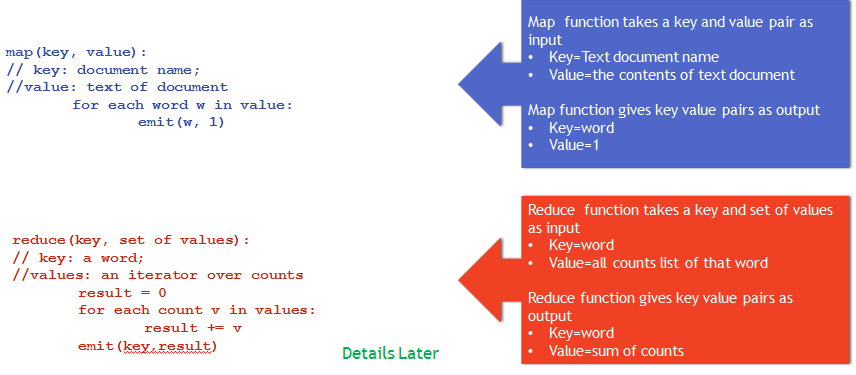### The Map Function input and output

• Input
• Map function takes a key and value pair as input
• Key = Text document name
• Value = the contents of text document
• Output
• Map function gives key value pairs as output
• Key = word
• Value = 1

### Map Reduce Word Count Example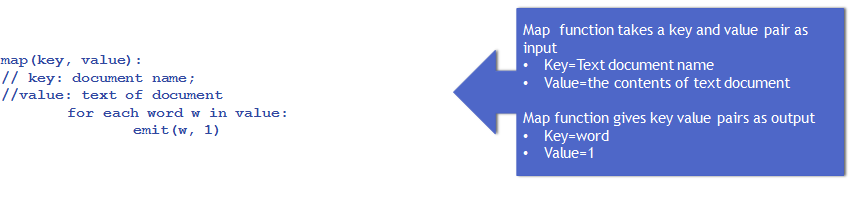### How Map Function Works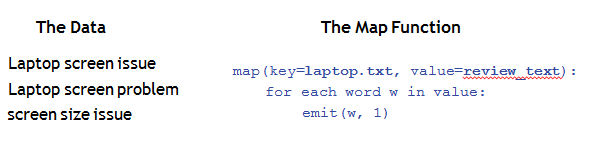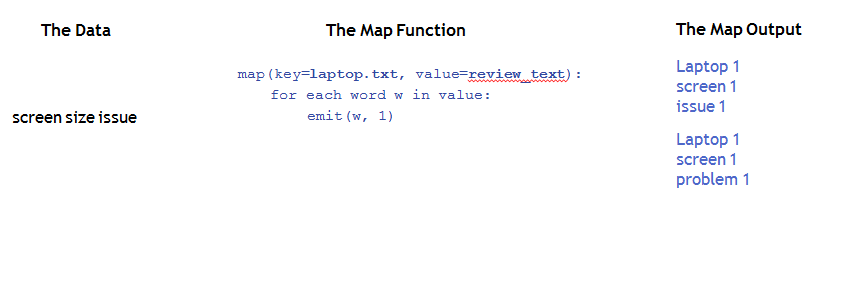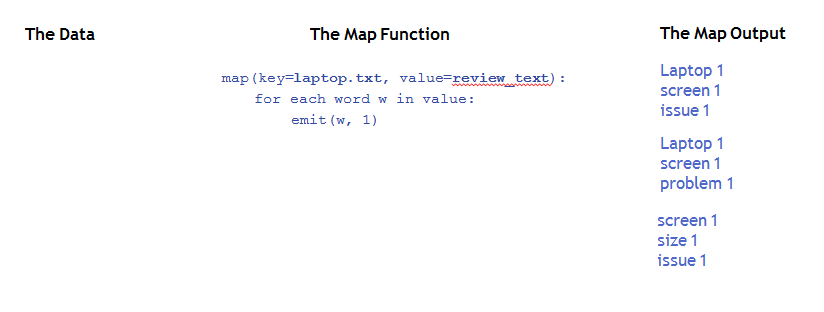### The Reduce Function input and output

• Input
• Reduce function takes a key and set of value pair as input
• Key=word
• Value=all counts list of that word
• Output
• Reduce function gives key value pairs as output
• Key=word
• Value=sum of counts

### How Reduce Function Works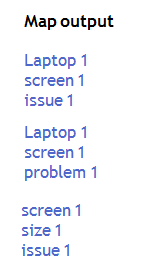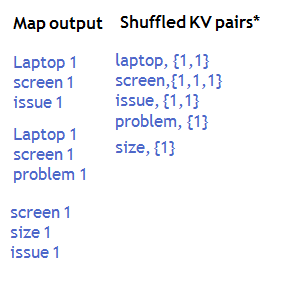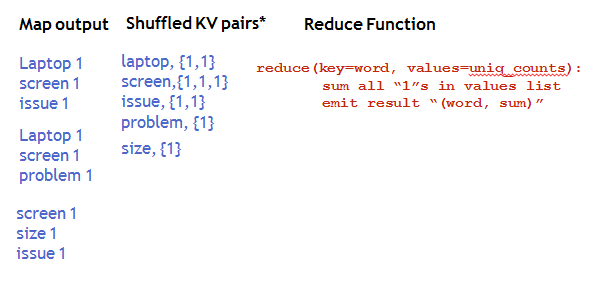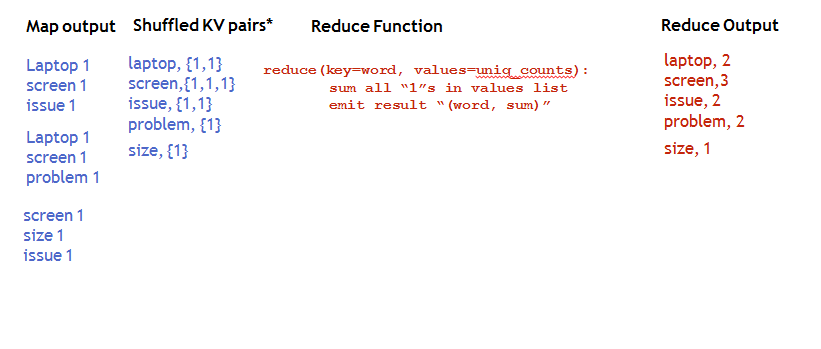22nd March 2018

### 0 responses on "301.2.6-How Map Reduce Works"

Statinfer Software Solutions LLP

Software Technology Parks of India,
NH16, Krishna Nagar, Benz Circle,